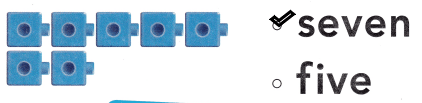# Texas Go Math Kindergarten Lesson 4.4 Answer Key Count and Write 7

Refer to our Texas Go Math Kindergarten Answer Key Pdf to score good marks in the exams. Test yourself by practicing the problems from Texas Go Math Kindergarten Lesson 4.4 Answer Key Count and Write 7.

## Texas Go Math Kindergarten Lesson 4.4 Answer Key Count and Write 7

Explore

DIRECTIONS: Count and tell how many cubes. Trace and write the numbers. Count and tell how many hats. Trace the word.Explanation:
I traced and practiced writing the number 7.I traced the word seven.

Share and Show

DIRECTIONS: 1. Look at the picture. Circle the sets of seven objects.

Question 1.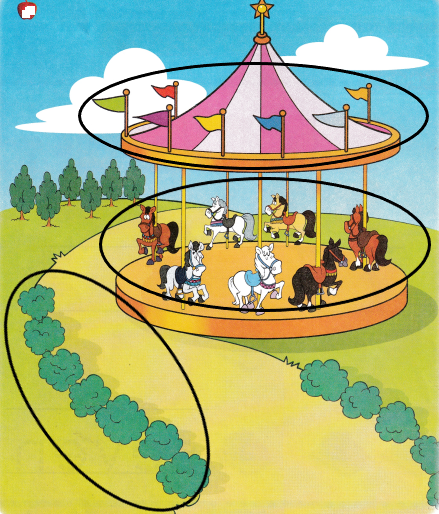Explanation:
I circled the objects that are 7 in number.There are 7 flags, hirses and bushes.

DIRECTIONS: 2. Say the number. Practice writing the number. 3-6. Count and tell how many. Write the number.

Question 2.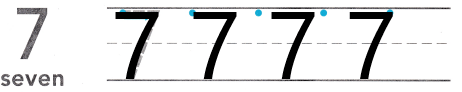Explanation:
I traced and practiced writing the number 7.

Question 3.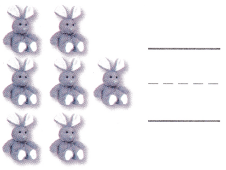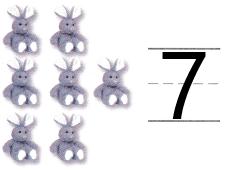Explanation:
There are 7 teddy’s in the image.So, i counted and wrote the number 7.

Question 4.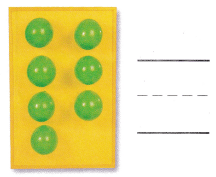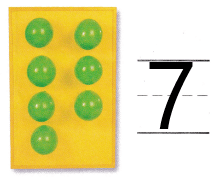Explanation:
There are 7 buttons in the image.So, i counted and wrote the number 7.

Question 5.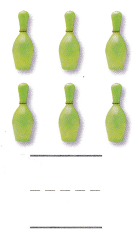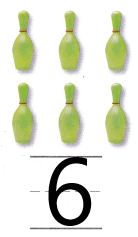Explanation:
There are 7 pins in the image.So, i counted and wrote the number 7.

Question 6.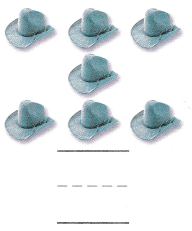Explanation:
There are 7 hats in the image.So, i counted and wrote the number 7.

HOME ACTIVITY • Show your child seven objects. Have him or her point to each object as he or she counts it. Then have him or her write the number on paper to show how many objects.

DIRECTIONS: 7. Jason has a number of ducks that is two greater than 5. Count the ducks in each set. Circle the set that shows a number of ducks that is two greater than 5. 8. Choose the correct answer. Which number does the model show?

Problem Solving

Question 7.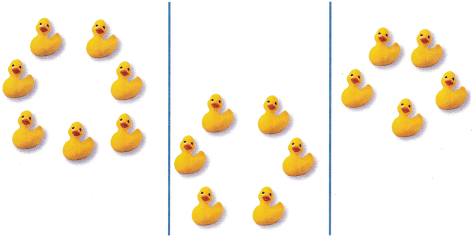Explanation:
I circled the group of ducks that are 7 in number as 2 greater than 5 is 7.

Question 8.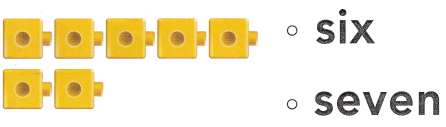Explanation:
I counted the number of cubes and marked the number 7 as there are 7 cubes in the image.

### Texas Go Math Kindergarten Lesson 4.4 Homework and Practice Answer Key

DIRECTIONS: 1-4. Count and tell how many. Write the number.

Question 1.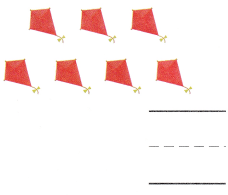Explanation:
There are 7 kites in the image.So, i counted and wrote the number 7.

Question 2.Explanation:
There are 6 aeroplanes in the image.So, i counted and wrote the number 6.

Question 3.Explanation:
There are 7 boats in the image.So, i counted and wrote the number 7.

Question 4.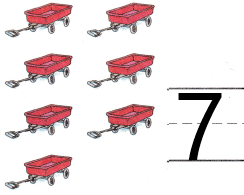Explanation:
There are 7 trollyes in the image.So, i counted and wrote the number 7.

DIRECTIONS: Choose the correct answer. 5. Which set has seven starfish? 6. Which set has seven ladybugs? 7. Which number does the model show?

Lesson Check

Question 5.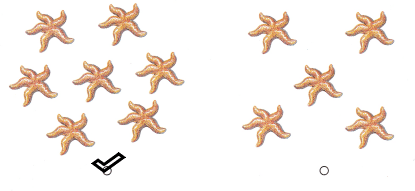Explanation:
I marked the group that has 7 starsfishes.

Question 6.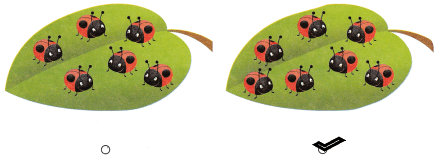Explanation:
I marked the group that has 7 ladybugs.

Question 7.